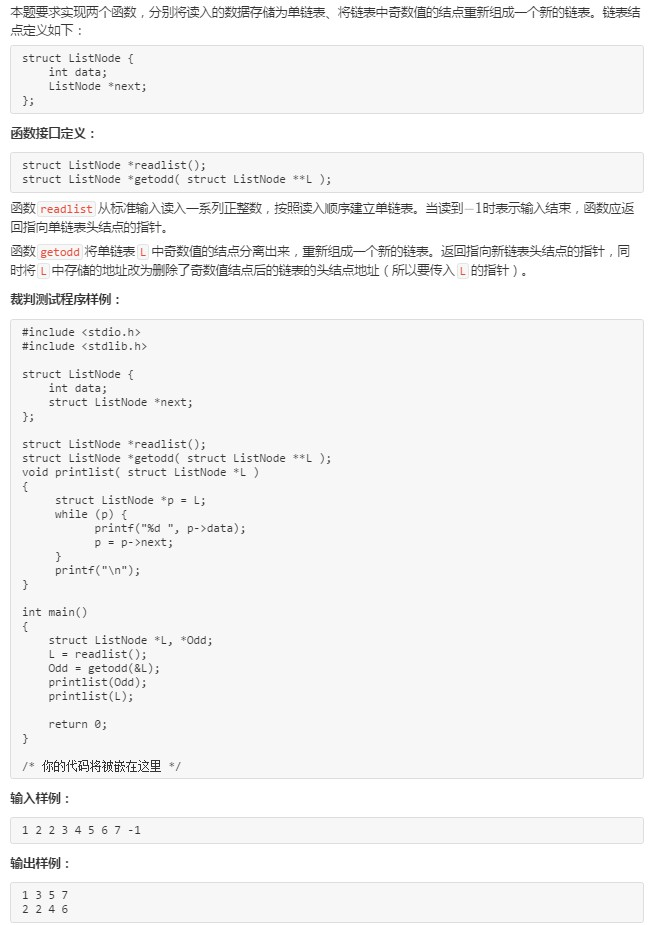{
int t=0;
scanf("%d",&t);
int size=sizeof(struct ListNode);
while(t!=-1)
{
p=(struct ListNode *)malloc(size);
p->data=t;
{
}else{
tail->next=p;
}
tail=p;
scanf("%d",&t);
}
tail->next=NULL;
}
struct ListNode *getodd( struct ListNode **L )
{
p=*L;
while(p!=NULL)
{
if(p->data%2!=0)
{
{
}else{
tail->next=p;
}
tail=p;
p=p->next;
}else{
{
}else{
tail2->next=p;
}
tail2=p;
p=p->next;
}
}
tail->next=NULL;
tail2->next=NULL;
}

2个回答

tail->next=NULL;
tail2->next=NULL;qh_45224223 我也想知道为什么这样就可以了，大佬能不能解释一下